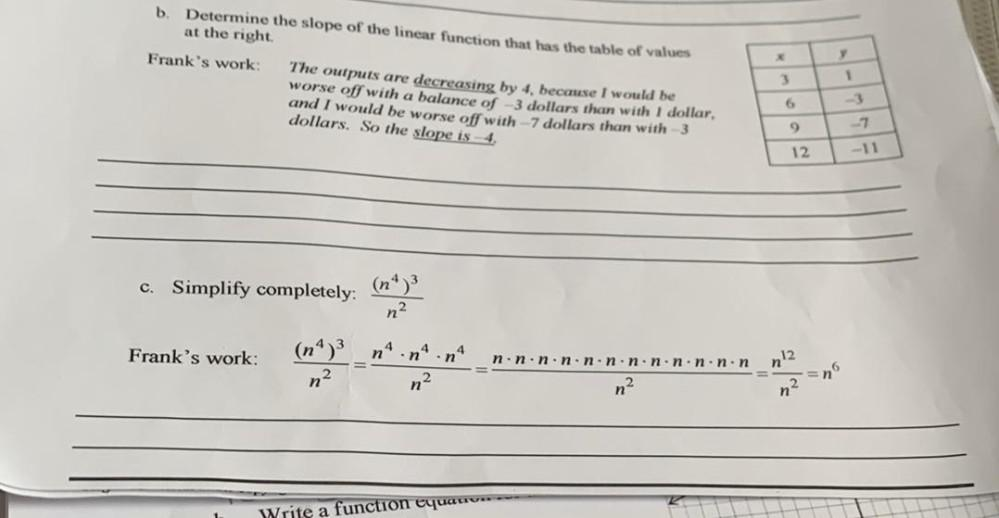Question:

# b Determine the slope of the linear function that has the table of values at the right Frank's work: The outputs are decreasingb Determine the slope of the linear function that has the table of values at the right Frank's work: The outputs are decreasing by 4. because I would be worse off with a balance of 3 dollars than with 1 dollar, and I would be worse off with -7 dollars than with 3 dollars. So the slope is 4. 3 1 6 9 -7 12 -11 c. Simplify completely: n2 Frank's work: 4 n n nº 12. =n n.n.n.n.non.n.n.n.n.non n- 2. n n? n Write a function equation-